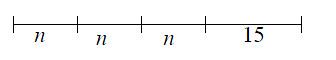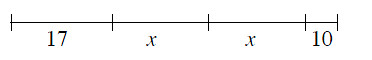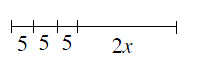### Home > MC1 > Chapter 9 > Lesson 9.1.2 > Problem9-18

9-18.

Write an algebraic expression to represent the length of each segment shown below.

1.$n + n + n + 15$ or $3n + 15$

2.$17 + x + x + 10$
Can you find a simpler way to write this expression?

3.See parts (a) and (b).